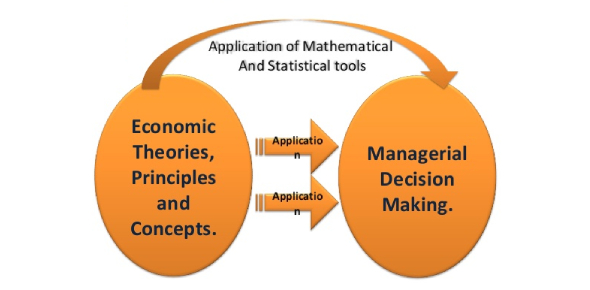# Managerial Economics Quiz Exam!

10 Questions | Total Attempts: 314Settings.

• 1.
Opportunity cost is not zero ______________
• A.

During general unemployment

• B.

Sunk costs

• C.

Free goods

• D.

When a machine has multiple uses

• E.

Full employement

• 2.
PPC curve is convex to the orgin:
• A.

Decreasing marginal rate of substitution

• B.

Constant marginal rate of substitution

• C.

Increasing marginal rate of substitution

• D.

Because of specialised resources

• E.

Maximum quantity produced at increasing cost

• 3.
Reservation price refers to?
• A.

Maximum price

• B.

Minimum price

• C.

Price floor

• D.

Price ceiling

• E.

Seller's price

• 4.
Price floor
• A.

• B.

Meant for sellers

• C.

• D.

Set by the government

• E.

Set on the floor

• 5.
Excess supply happens when there is:
• A.

Price floor

• B.

Rent control

• C.

Consumer surplus

• D.

Due to govt subsidies

• E.

Due to govt taxes

• 6.
When income of the consumer increases the demand for Giffen good:
• A.

Goes up

• B.

Goes down

• C.

Depends up on the price of the good

• D.

Constant

• E.

It goes up certain level then remain constant

• 7.
• A.

Loss of social welfare

• B.

Loss of sellers

• C.

• D.

Excess demand

• E.

Excess supply

• 8.
Which of the following are not determinants of supply?
• A.

Income of the consumer

• B.

Price of substitues

• C.

Infrastructure

• D.

Cost of inputs

• E.

Price of complements

• 9.
If the price of the commodity tends to high then it enjoys:
• A.

Elastic demand

• B.

Inelastic demand

• C.

Unit elastic

• D.

Perfectly inelastic demand

• E.

Perfectly elastic demand

• 10.
Which of the following statement is false?
• A.

Price line is required to draw consumer's equilibrium under IC analysis

• B.

The consumer is hooked to highest possible IC under equilibrium

• C.

Under price ceiling hoarding and black marketing take place

• D.

Wider the substitute for a good higher elasticity of demand for a good

• E.

The MU of money cannot remain constant

Related TopicsBack to top